### Home > CALC > Chapter 9 > Lesson 9.4.2 > Problem9-127

9-127.
1. Suppose that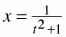and y = t2 for all real t. Homework Help ✎

1. Express x as a function of y.

2. Express y as a function of x.

3. How would the graphs of the parametric equations given above be different if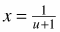and y = u for all real u?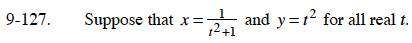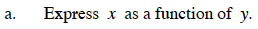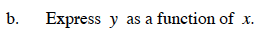Solve your equation from part (a) for y.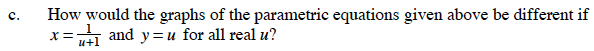What are the possible values of x and y if: